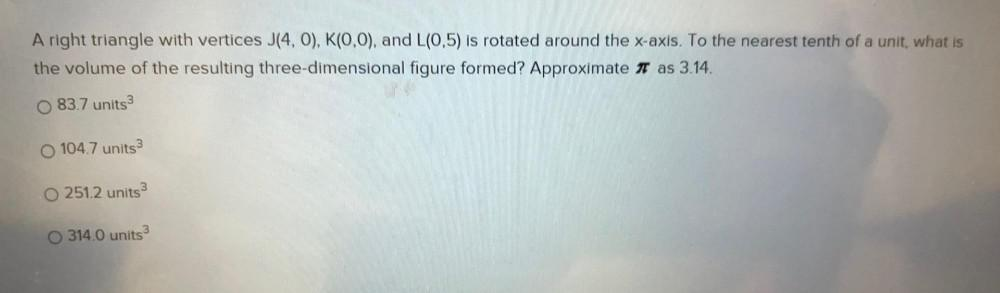Question:

# A right triangle with vertices J(4,0), K(0,0), and L(0,5) is rotated around the x-axis. To the nearest tenth of a unit, what isA right triangle with vertices J(4,0), K(0,0), and L(0,5) is rotated around the x-axis. To the nearest tenth of a unit, what is the volume of the resulting three-dimensional figure formed? Approximate it as 3.14. 83.7 units 3 O 104.7 units 3 O 251.2 units 3 314.0 units